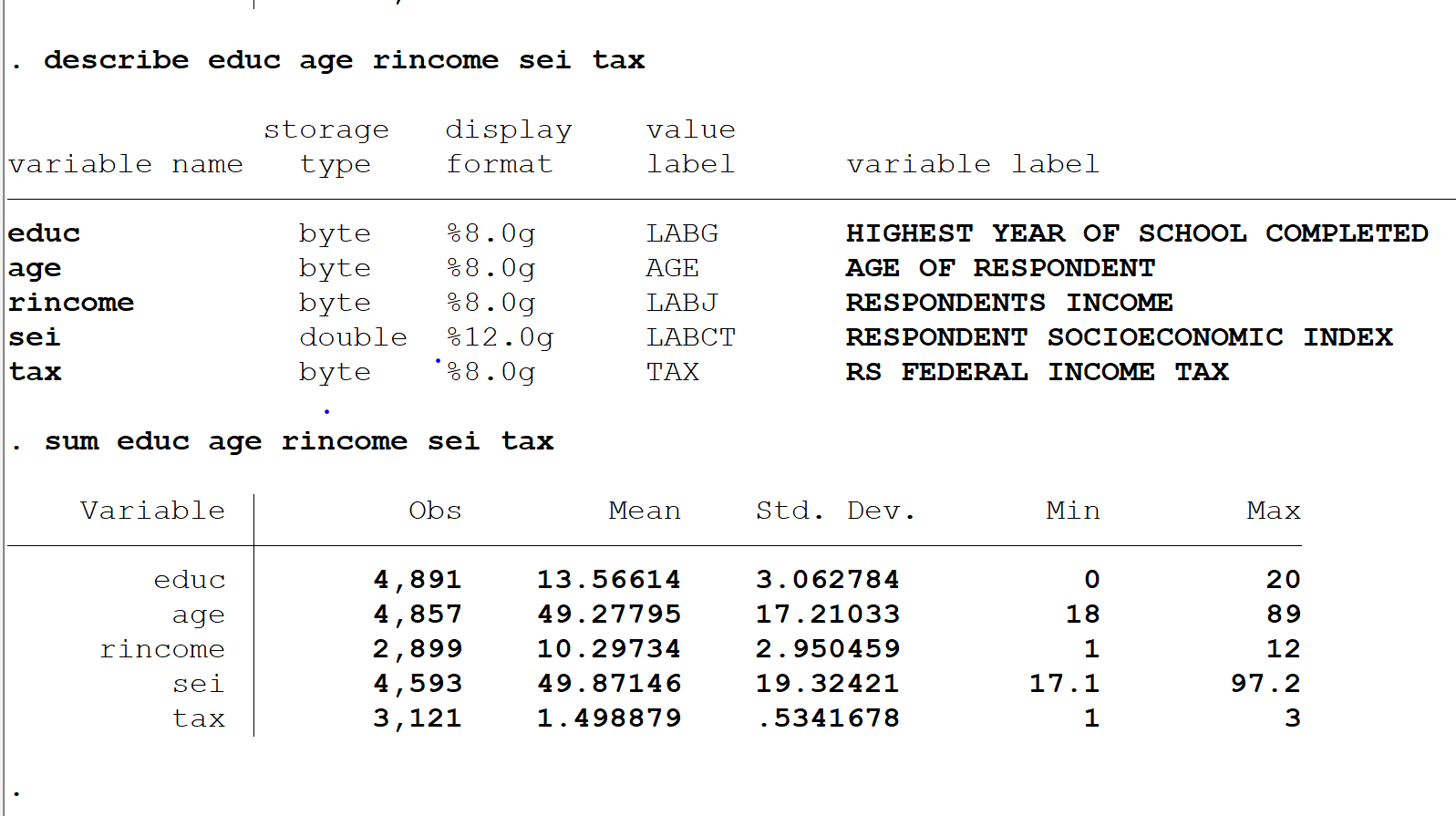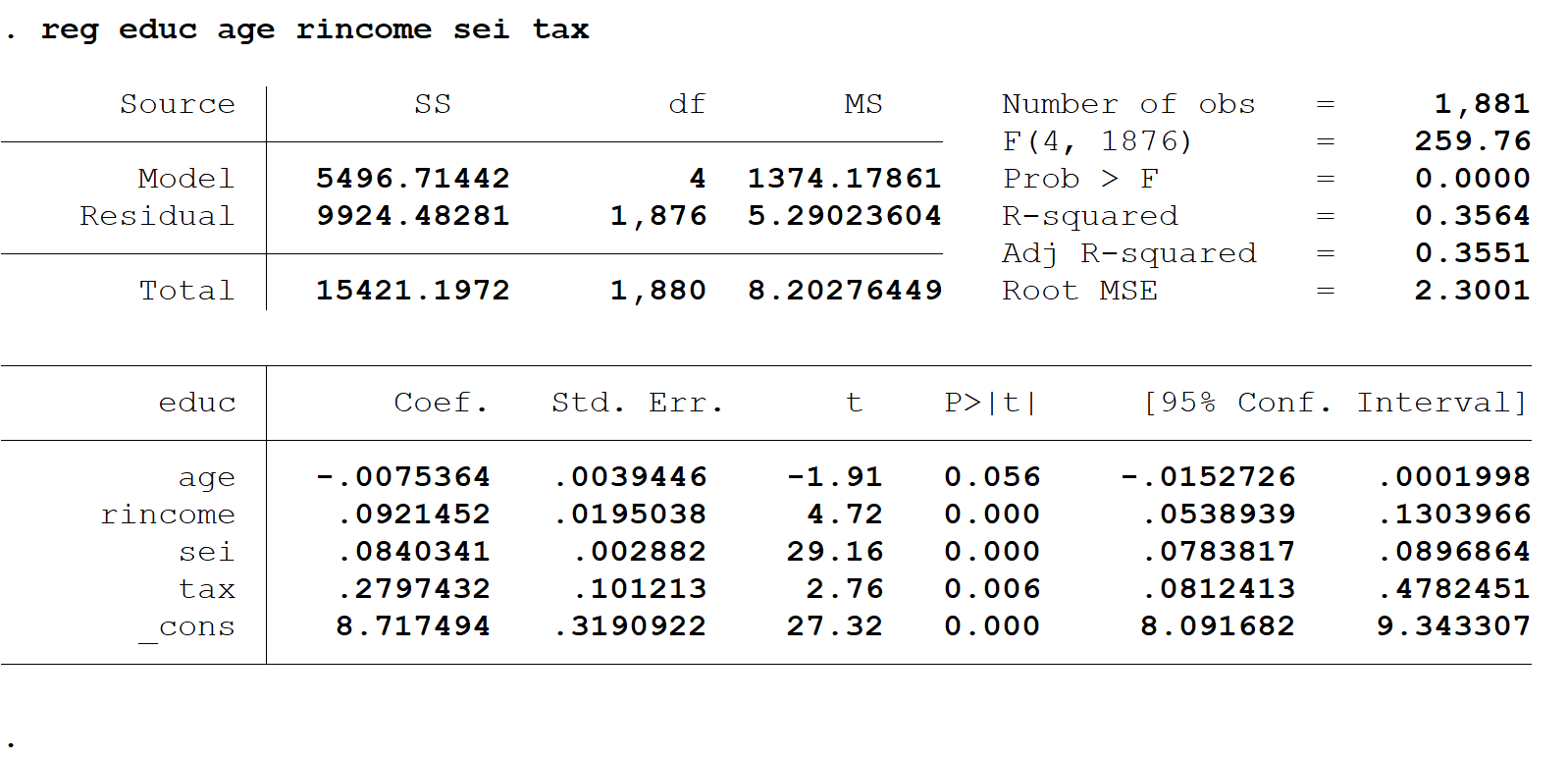# Interpretation of empirical results

 Interpretation of empirical results This part gives information about empirical results that are obtained from STATA program. First of all, variables in that model are described and summarized.In the STATA Information above, Observation, Mean, Standard deviation, minimum and maximum of each variable are illustrated.This regression gives information about variables and p-value, coefficient of each variables, t-value and R­2. Regression analysis is that F(4,1876)=259.76 Prob>F=0.0000. R2=0.3551 analyses that in regression 2.35% of education explain Xi variable model when 97.65% illustrate disturbance Ui. When p-value>0.05 shows indicators insignificant and in this case age is insignificant and other variables are significant that are family income, Socioeconomic and tax. H0: β1=0=β2=0=β3=0 Ha: At least one of the Betas is not equal to zero Higher education = 8.717494 + 0.0921452*income + 0.0075364*age + 0.0840341* socioeconomic + 0.2797432* tax+ Ui β0=8.717494 β 1=0.0921452 β 2=0.0075364 β 3=0.0840341 β 4=0.2797432 Download 307.32 Kb.Do'stlaringiz bilan baham: